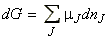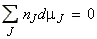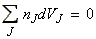Back to Table of Contents [TOP] 5. Chemical potential, simple mixtures, chemical reactions andequilibria  (ii) Mixtures The Gibbs-Duhem equation Since the total free energy of a system is give by:and hence by differentiation we get:But, as discussed in the previous section, for a system at constant temperature and pressure we have:and hence since G is a state function, then the two expressions must be equal, which means that:.(Gibbs-Duhem equation) The significance of the Gibbs-Duhem equation is that the chemical potential of a component in a mixture cannot change independently of the chemical potential of the other components. For example, in a binary mixture we have:i.e., if one chemical potential increases, the other must decrease.   Note: There is also the a similar 'Gibbs-Duhem equation for the partial molar volumes' which is given by:where the partial molar volume, defined as:which signifies the volume of component J in a mixture.